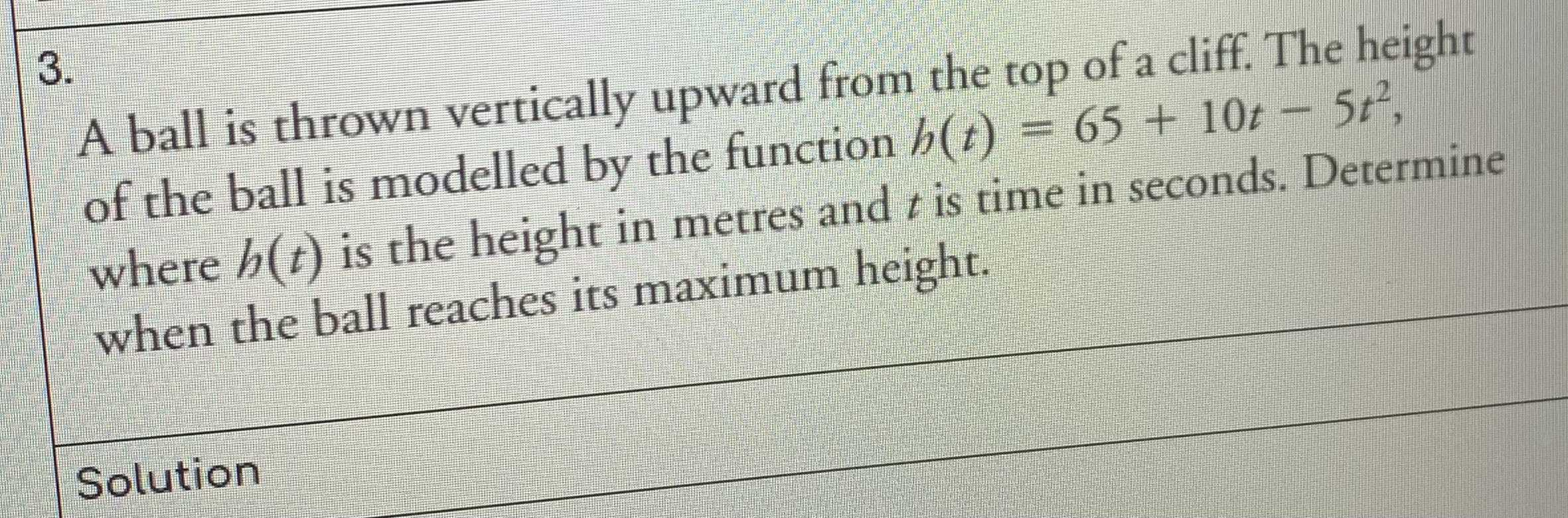### ¿Todavía tienes preguntas de matemáticas?

Pregunte a nuestros tutores expertos
Algebra
Pregunta3. A ball is thrown vertically upward from the top of a cliff. The height of the ball is modelled by the function $$h ( t ) = 65 + 10 t - 5 t ^ { 2 }$$ , where $$h ( t )$$ is the height in metres and $$t$$ is time in seconds. Determine when the ball reaches its maximum height.